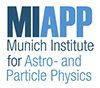#Exploring the Perfect Liquid

Sep 6 – 8, 2018
Max Planck Institute for Astrophysics (MPA)
Europe/Berlin timezone

## Measurements of heavy-flavour correlations and jets with ALICE

Sep 7, 2018, 4:35 PM
20m
E.0.11 (Max Planck Institute for Astrophysics (MPA))

### E.0.11

#### Max Planck Institute for Astrophysics (MPA)

Karl-Schwarzschild-Str. 1 85748 Garching
Open Heavy Flavour

### Speaker

Barbara Trzeciak (Utrecht University)

### Description

This contribution will focus on the latest heavy-flavour correlation and jet measurements with the ALICE detector in pp, p--Pb and Pb--Pb collisions at the LHC.
In particular, the results of azimuthal correlations of D mesons with charged particles in pp collisions at $\sqrt{s}=$ 7 and 13 TeV and in p--Pb collisions at $\sqrt{s_{\rm NN}}=$ 5.02 TeV will be presented. Measurements of multiplicity and centrality dependent azimuthal correlations of heavy-flavour hadron decay electrons with charged particles in p--Pb and Pb--Pb collisions at $\sqrt{s_{\rm NN}}=$ 5.02 TeV will be shown together with the heavy-flavour electron $v_{2}$ in p--Pb collisions.
Furthermore, measurements of D-meson tagged jet production in pp collisions at $\sqrt{s}=$ 7 TeV including studies of the jet-momentum fraction carried by the D meson will be presented. The recent results in p--Pb collisions at $\sqrt{s_{\rm NN}}=$5.02 TeV will be reported. The first measurement of the nuclear modification factor of D-tagged jet in Pb--Pb collisions at $\sqrt{s_{\rm NN}}=$ 5.02 TeV will be also discussed.

### Primary authors

Barbara Trzeciak (Utrecht University)